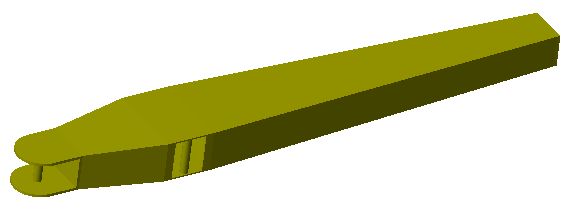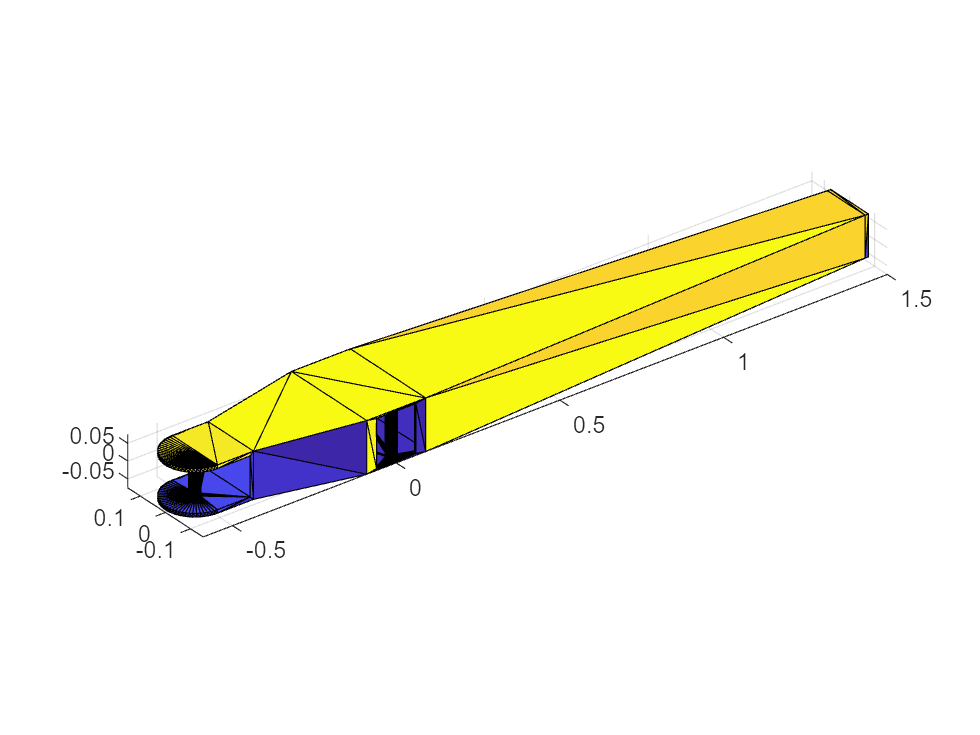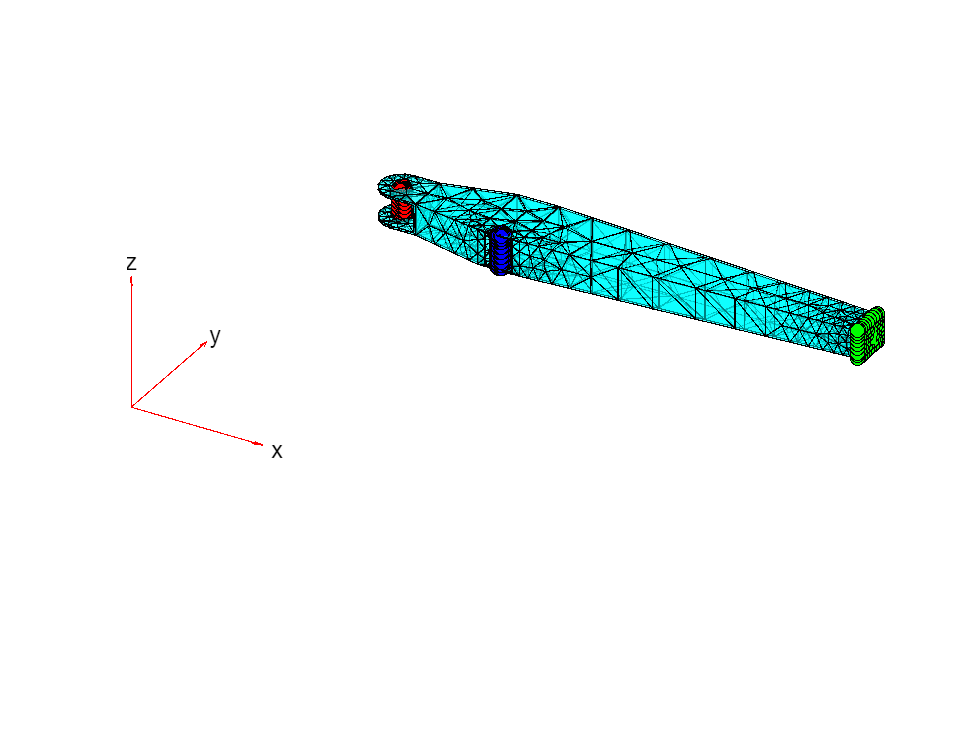# Model an Excavator Dipper Arm as a Flexible Body

The Reduced Order Flexible Solid block models a deformable body based on a reduced-order model that characterizes the geometric and mechanical properties of the body. The basic data imported from the reduced-order model includes:

• A list of coordinate triples that specify the position of all interface frame origins relative to a common reference frame.

• A symmetric stiffness matrix that describes the elastic properties of the flexible body.

• A symmetric mass matrix that describes the inertial properties of the flexible body.

There are several ways to generate the reduced-order data required by this block. Typically, you generate a substructure (or superelement) by using finite-element analysis (FEA) tools.

This example uses the Partial Differential Equation Toolbox™ to create a reduced-order model for a flexible dipper arm, such as the arm for an excavator or a backhoe. You start with the CAD geometry of the dipper arm, generate a finite-element mesh, apply the Craig-Bampton FEA substructuring method, and generate a reduced-order model. The model `sm_flexible_dipper_arm` uses the reduced-order data from this example. In the model, the dipper arm is mounted on top of a rotating tower as part of a test rig. For more information, see Flexible Dipper Arm.### Step 1: Define the Geometry and Material Properties of the Dipper Arm

The file `sm_flexible_dipper_arm.STL` contains a triangulation that defines the CAD geometry of the dipper arm. To view the geometry stored in this file, use the MATLAB® functions `stlread` and `trisurf`:

```stlFile = 'sm_flexible_dipper_arm.STL'; figure trisurf(stlread(stlFile)) axis equal```The dipper arm is constructed from steel. To represent its material properties, set these values for Young's modulus, Poisson's ratio, and mass density:

```E = 200e9; % Young's modulus in Pa nu = 0.26; % Poisson's ratio (nondimensional) rho = 7800; % Mass density in kg/m^3```

### Step 2: Specify the Locations of Interface Frames

The dipper arm has three interface frames where you can connect other Simscape™ Multibody™ elements, such as joints, constraints, forces, and sensors:

• The cylinder connection point, where the arm connects to a hydraulic cylinder that actuates the arm vertically.

• The bucket connection point, where the arm connects to the excavator bucket.

• The fulcrum point, where the arm connects to the excavator boom.

The positions of all interface frame origins are specified in meters relative to same common reference frame used by the CAD geometry.

```origins = [-0.500 0 0 % Frame 1: Cylinder connection point 1.500 0 0 % Frame 2: Bucket connection point 0 -0.130 0]; % Frame 3: Fulcrum point numFrames = size(origins,1);```

### Step 3: Create the Finite-Element Mesh

To generate the mesh for the dipper arm, first call the `createpde` function, which creates a structural model for modal analysis of a solid (3-D) problem. After importing the geometry and material properties of the arm, the `generateMesh` function creates the mesh.

```feModel = createpde('structural','modal-solid'); importGeometry(feModel,stlFile); structuralProperties(feModel, ... 'YoungsModulus',E, ... 'PoissonsRatio',nu, ... 'MassDensity',rho); generateMesh(feModel, ... 'GeometricOrder','quadratic', ... 'Hmax',0.2, ... 'Hmin',0.02);```

### Step 4: Set up the Multipoint Constraints for the Interface Frames

Each interface frame on the block corresponds to a boundary node that contributes six degrees of freedom to the reduced-order model. There are several ways to ensure that the FEA substructuring method preserves the required degrees of freedom. For example, you can create a rigid constraint to connect the boundary node to a subset of finite-element nodes on the body. You can also use structural elements, such as beam or shell elements, to introduce nodes with six degrees of freedom.

This example uses a multipoint constraint (MPC) to preserve the six degrees of freedom at each boundary node. To identify the geometric regions (such as faces, edges, or vertices) to associate with each MPC, first plot the arm geometry by using the function `pdegplot`:

```figure pdegplot(feModel,'FaceLabels','on','FaceAlpha',0.5)```You can zoom, rotate, and pan this image to determine the labels for the faces corresponding to the boundary nodes. These faces define the MPCs associated with the boundary nodes in the dipper arm:

• Cylinder connection point: face 1

• Bucket connection point: face 27

• Fulcrum point: face 23

`faceIDs = [1,27,23]; % List in the same order as the interface frame origins`

To verify these values, plot the mesh and highlight the selected faces:

```figure pdemesh(feModel,'FaceAlpha',0.5) hold on colors = ['rgb' repmat('k',1,numFrames-3)]; assert(numel(faceIDs) == numFrames); for k = 1:numFrames nodeIdxs = findNodes(feModel.Mesh,'region','Face',faceIDs(k)); scatter3( ... feModel.Mesh.Nodes(1,nodeIdxs), ... feModel.Mesh.Nodes(2,nodeIdxs), ... feModel.Mesh.Nodes(3,nodeIdxs), ... 'ok','MarkerFaceColor',colors(k)) scatter3( ... origins(k,1), ... origins(k,2), ... origins(k,3), ... 80,colors(k),'filled','s') end hold off```Call the function `structuralBC` to define the MPCs for the boundary nodes in these faces:

```for k = 1:numFrames structuralBC(feModel, ... 'Face',faceIDs(k), ... 'Constraint','multipoint', ... 'Reference',origins(k,:)); end```

### Step 5: Generate the Reduced-Order Model

The function `reduce` applies the Craig-Bampton order reduction method and retains all fixed-interface modes up to a frequency of $1{0}^{4}$ radians per second.

`rom = reduce(feModel,'FrequencyRange',[0 1e4]);`

Store the results of the reduction in a data structure `arm`. Transpose the `ReferenceLocations` matrix to account for the different layout conventions used by Partial Differential Equation Toolbox and Simscape Multibody.

```arm.P = rom.ReferenceLocations'; % Interface frame locations (n x 3 matrix) arm.K = rom.K; % Reduced stiffness matrix arm.M = rom.M; % Reduced mass matrix```

The function `computeModalDampingMatrix`, which is defined at the bottom of this page, computes a reduced modal damping matrix with a damping ratio of 0.05:

```dampingRatio = 0.05; arm.C = computeModalDampingMatrix(dampingRatio,rom.K,rom.M);```

The boundary nodes in the reduced-order model must be specified in the same order as the corresponding interface frames on the block. This order is given by the rows of the array `origins`. If the order of the MPCs is different than the order specified by `origins`, permute the rows and columns of the various matrices so that they match the original order.

```frmPerm = zeros(numFrames,1); % Frame permutation vector dofPerm = 1:size(arm.K,1); % DOF permutation vector assert(size(arm.P,1) == numFrames); for i = 1:numFrames for j = 1:numFrames if isequal(arm.P(j,:),origins(i,:)) frmPerm(i) = j; dofPerm(6*(i-1)+(1:6)) = 6*(j-1)+(1:6); continue; end end end assert(numel(frmPerm) == numFrames); assert(numel(dofPerm) == size(arm.K,1)); arm.P = arm.P(frmPerm,:); arm.K = arm.K(dofPerm,:); arm.K = arm.K(:,dofPerm); arm.M = arm.M(dofPerm,:); arm.M = arm.M(:,dofPerm); arm.C = arm.C(dofPerm,:); arm.C = arm.C(:,dofPerm);```

### Step 6: Import Reduced-Order Data

The model `sm_flexible_dipper_arm` uses the data structure `arm` to set up the parameters of the Reduced Order Flexible Solid block. In the block, these parameters import the reduced-order data:

• Origins: `arm.P`

• Stiffness Matrix: `arm.K(1:24,1:24)`

• Mass Matrix: `arm.M(1:24,1:24)`

• Damping Matrix: `arm.C(1:24,1:24)`

### Compute Modal Damping Matrix Function

This function computes a modal damping matrix associated with the stiffness matrix `K` and mass matrix `M`. This function applies a single scalar damping ratio to all of the flexible (non-rigid-body) normal modes associated with `K` and `M`.

```function C = computeModalDampingMatrix(dampingRatio,K,M) % To avoid numerical issues (such as complex eigenvalues with very small % imaginary parts), make the matrices exactly symmetric. K = (K+K')/2; % Stiffness matrix M = (M+M')/2; % Mass matrix % Compute the eigen-decomposition associated with the mass and stiffness % matrices, sorting the eigenvalues in ascending order and permuting % the corresponding eigenvectors. [V,D] = eig(K,M); [d,sortIdxs] = sort(diag(D)); V = V(:,sortIdxs); % Due to small numerical errors, the six eigenvalues associated with the % rigid-body modes may not be exactly zero. To avoid numerical issues, % check that the first six eigenvalues are close enough to zero. Then % replace them with exact 0 values. assert(all(abs(d(1:6))/abs(d(7)) < 1e-9),'Error due to "zero" eigenvalues.'); d(1:6) = 0; % Vectors of generalized masses and natural frequencies MV = M*V; generalizedMasses = diag(V'*MV); naturalFrequencies = sqrt(d); % Compute the modal damping matrix associated with K and M C = MV * diag(2*dampingRatio*naturalFrequencies./generalizedMasses) * MV'; end```